## Recent trends and mutual information-based objectives in unsupervised learning

#### August 2019

Supervised learning is definitely the most popular form of machine learning, but is often prohibited in practice due to lack of available labelled data. For this reason, unsupervised learning has presented itself as a solution since it doesn't require data to be annotated and thus large unlabelled datasets are ready for use.

A large component of unsupervised learning is trying to compress data by extracting useful information that ultimately makes it easier for classifiers and other predictors to perform downstream tasks on the compressed version. This is referred to as representation learning, and the representations should ideally capture valuable information in the data that any classifier or predictor would find useful (referred to as signal) and thus ignore noise which is not helpful. For example, a convolutional neural network trained to perform image classification builds a representation by successively performing convolution (and other) operations that its softmax classifier can easily tag as cat, dog, or some other thing. Similarly, many natural language processing tasks rely on word vectors--a representation of each word in a vocabulary which models its distributional semantics using a metric space.

This post will cover how mutual information, a sort of metric grounded in information theory, can be used to compress data and extract desirable representations that are helpful for downstream tasks.

#### Mutual Information

Mutual information is growing in popularity as an objective function in unsupervised learning--let's first dissect what it is. Mutual information measures how informative one variable is of another variable. For example, if $$x$$ measures how long you will wait for the bus this morning and $$z$$ measures the outcome of rolling one die, then the mutual information between $$x$$ and $$z$$, denoted as $$I(x; z)$$ is zero. Mathematically,

\begin{align*} I(x; z) &= H(x) - H(x|z) \\ &= H(z) - H(z|x) \\ \end{align*}

where $$H(\cdot)$$ is called the entropy and measures the uncertainty about a variable, whereas $$H(\cdot | \cdot)$$ is called the conditional entropy and measures the uncertainly about a variable given information about the other. Intuitively, you can think of mutual information as the"decrease in uncertainty" about one variable given some knowledge about the other. So if $$H(x)$$ measures the uncertainty about how long you'll wait for the bus this morning, then $$H(x|z)$$ is the same as $$H(x)$$ since the uncertainty about how long you'll have to wait won't decrease by knowing the outcome of rolling a die (which is totally random), hence $$I(x; z) = 0$$!

Mutual information is also defined as a Kullback-Leibler (KL) divergence between the joint distribution of $$x$$ and $$z$$ and the product of their marginal distributions. The marginal distribution of $$x$$, given as $$p(x)$$, gives the probability of a particular value of observing $$x$$ (regardless of $$z$$, and the same is true of $$p(z)$$), whereas the joint distribution between the two variables $$p(x, z)$$ gives the probability of seeing each $$x$$ and $$z$$.

As we'll soon see, this formulation is useful in machine learning, but let's quickly review what a KL-divergence is. The KL-divergence for two probability distributions $$q_\text{A}(x)$$ and $$q_\text{B}(x)$$ intuitively measures how different they are, and is defined as

$D_\text{KL}\left( q_\text{A}(x) || q_\text{B}(x) \right) = \mathbb{E}_{q_\text{A}(x)} \left[ \log \frac{q_\text{A}(x)}{q_\text{B}(x)} \right].$

Since it's some sort of a distance measurement, KL-divergences are always non-negative (remember this!). If the two distributions are the same for all values of $$x$$ (i.e., they have "distance" zero), then the ratio $$q_\text{A}(x) / q_\text{B}(x)$$ is always 1, hence $$D_\text{KL}\left( q_\text{A}(x) || q_\text{B}(x) \right) = 0$$ and the same is true vice-versa.

Now that we've added KL-divergences to our toolkit, we can proceed! Mutual information can be written as the KL-divergence between $$p(x, z)$$ and $$p(x) p(z)$$:

$I(x; z) = D_\text{KL} \left( p(x, z) || p(x) p(z) \right).$

Recall from you first stats class that if $$x$$ and $$z$$ are independent, then $$p(x, z) = p(x) p(z)$$, hence $$D_\text{KL} \left( p(x, z) || p(x) p(z) \right) = 0$$ and hence their mutual information is zero.

Okay, now we know what mutual information is--great! In the context of representation learning, $$x$$ is the data which needs to be compressed and $$z$$ its learned representation. To induce a high correlation between $$x$$ and $$z$$, an unsupervised learning objective is simply to maximize the $$I(x; z)$$ so that $$z$$ maximally encodes information about $$x$$. Based on the second definition of mutual information, we want to maximize the KL-divergence between $$p(x, z)$$ and $$p(x) p(z)$$ because driving these two distributions apart makes $$x$$ and $$z$$ less and less independent of each other. Pretty simple, right?

Not entirely. In machine learning, the data dimension is typically in the order of hundreds or even thousands, and mutual information is extremely difficult to calculate in high dimensions, especially when the distributions $$p(x, z)$$, $$p(x)$$, or $$p(z)$$ are not explicitly known. For instance, as we will soon see, if $$x$$ represents a set of images (where the dimension of the space is $$\text{total number pixels} \times 3$$ for RGB color channels), then there's no easy way to approximate the distribution $$p(x)$$. Sure, we can sample images at random to get a sense of the mean and variance across each dimension, but we would need to explicitly assume the family of distribution (e.g., Gaussian) in addition to incurring a large sampling cost due to the number of dimensions. In other words, estimating these distributions in quite infeasible.

Okay, so we can't explicitly compute mutual information, now what? To sidestep explicit computation of mutual information, we can generally find an easy-to-compute lower bound on $$I(x; z)$$ which can be evaluated, differentiated, and thus maximized with gradient-based methods that we're already familiar with. In this post, I will discuss three such methods have been employed successfully in the recent past, and all of them find a lower bound on mutual information to maximize. Let's get started!

#### 1. Variational Information Maximization

As discussed, mutual information can be decomposed into a difference of entropy terms: $$I(x; z) = H(z) - H(z|x)$$. Using this formulation, we can derive a variational lower bound, which is a lower bound on $$I(x; z)$$ as a function of a probability distribution $$q_\theta(z|x)$$ that we can explicitly parameterize. For the following derivation, its worth noting that $$H(z|x) = -\mathbb{E}_{p(x, z)} \left[ \log p(x, z) - \log p(x) \right]$$.

\begin{align*} I(x; z) &= H(z) - H(z|x) \\ &= H(z) + \mathbb{E}_{p(x, z)} \left[ \log \frac{p(x, z)}{p(x)} \right] \\ &= H(z) + \mathbb{E}_{p(x)} \left[ \mathbb{E}_{p(z|x)} \left[ \log p(z|x) \right] \right] \\ &= H(z) + \mathbb{E}_{p(x)} \left[ \mathbb{E}_{p(z|x)} \left[ \log \frac{p(z|x)}{q_\theta(z|x)} \right] + \mathbb{E}_{p(z|x)} \left[ \log q_\theta(z|x) \right] \right] \\ &= H(z) + \mathbb{E}_{p(x)} \bigg[ \underbrace{ D_\text{KL}\left( p(z|x) || q_\theta(z|x) \right) }_{\geq 0} + \mathbb{E}_{p(z|x)} \left[ \log q_\theta(z|x) \right] \bigg] \\ &\geq H(z) + \mathbb{E}_{p(x)} \left[ \mathbb{E}_{p(z|x)} \left[ \log q_\theta(z|x) \right] \right], \end{align*}

where the inequality holds since KL-divergences are always non-negative. We can now choose $$q_\theta$$ to be a neural network with parameters $$\theta$$ and easily maximize the variational lower bound lower bound via backpropagation.

This approach is leveraged by Information Maximizing Generative Adversarial Network (or InfoGAN, for short) developed by a team of researchers at UC Berkeley and OpenAI. Generative Adversarial Networks (GANs, don't worry about the details) try to model the data distribution $$p(x)$$ by mapping a randomly chosen representation (or "latent code") $$c$$ to a sample $$x$$. However, the latent features usually don't correspond to any particular semantic features in the data. InfoGAN, on the other hand, can control for certain semantic features by inducing high mutual information between $$x$$ and $$c$$. This means that if $$p(x)$$ is the distribution over images of human faces, one dimension of $$c$$ could correspond to eye color, another dimension to cheekbone height, etc.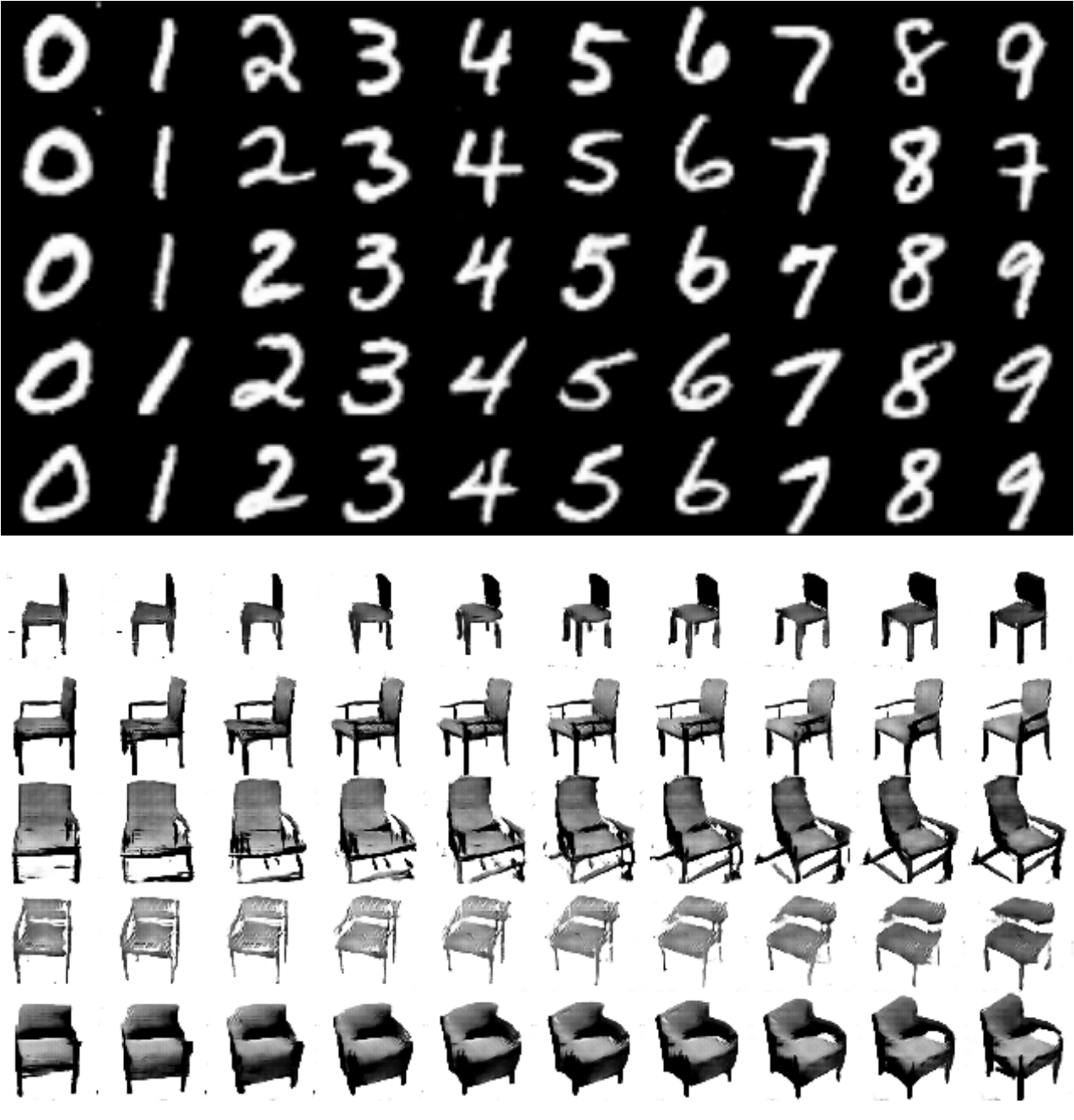Disentangling factors of variation using InfoGAN. Top: when generating handwritten digits from the MNIST dataset, a certain code in the representation can control which digit is generated. Bottom: A certain latent code can be used to control for rotation when generating images of chairs. Source: Chen et al. (2016).

As a footnote, Deep Variational Information Bottleneck (developed by Google AI) uses a similar idea, but the objective there is to find the optimal data compression in a supervised setting by maximizing mutual information between representation and decoder output.

#### 2. Contrastive Lower Bound

A contrastive objective can also be used as a lower bound on mutual information, as shown just last year by researchers at DeepMind. The main idea behind a contrastive objective is that "a good model should be able to tell apart noise from data". But what does this mean in the context of representation learning? if $$(x, z)$$ forms a data-representation pair drawn from $$p(x, z)$$ and $$x'$$ is some other data point (e.g., some other image) from $$p(x)$$, then a model should be able to somehow know that $$(x, z)$$ was drawn from the joint distribution $$p(x, z)$$ while $$(x', z)$$ was drawn from the product of marginals $$p(x) p(z)$$. In essence, the model learning to contrast samples drawn from $$p(x, z)$$ versus $$p(x) p(z)$$.

Let's call that model $$h_\theta$$, parameterized once again by a neural network with parameters $$\theta$$, and it outputs a real number. $$h_\theta$$'s goal is to estimate the ratio $$p(x, z) / p(x) p(z)$$. How does it do this? By optimizing

$\max_\theta \mathbb{E}_S \left[ \log \frac{ h_\theta(x^*, z^*) }{ \sum_{x \in S} h_\theta(x, z^*) } \right],$

where $$(x^*, z^*)$$ is a data-representation pair from $$p(x, z)$$ and $$S = \{x^*, x_1, \ldots, x_{N-1}\}$$ is a set of data points, $$N-1$$ of which are negative samples. Consequently, the $$(x_j, z^*)$$ pairs form pairs drawn from $$p(x) p(z)$$ where $$1 \leq j < N$$.

So what's going on here? By maximizing the above objective, $$h_\theta$$ is learning to output a relatively high value for $$(x^*, z^*)$$ and a relatively low value for $$(x_j, z^*)$$. It's thus learning to estimate the ratio $$p(x, z) / p(x) p(z)$$ as desired by contrasting the true data point $$x^*$$ that associates with representation $$z^*$$ with $$N-1$$ other data points that don't associate with $$x^*$$.

Okay, that's great, but how does this maximize mutual information between our data and learned representations? Below let's show that the contrastive objective above is a lower bound $$I(x; z) - \log N$$ and thus a lower bound $$I(x; z)$$. Also note that $$p(x^*, z^*) = p(x^* | z^*) p(z^*)$$ by Bayes rule.

\begin{align*} I(x; z) - \log N &= \mathbb{E}_S \left[ \log \frac{ p(x^*|z^*) }{ p(x^*) } \right] - \log N \\ &= \mathbb{E}_S \left[ \log \left( \frac{ p(x^*|z^*) }{ p(x^*) } \frac{1}{N} \right) \right] \\ &= \mathbb{E}_S \left[ \log \left( \frac{ 1 }{ \frac{p(x^*)}{p(x^*|z^*)} } \frac{1}{N} \right) \right] \\ &\geq \mathbb{E}_S \left[ \log \frac{1}{ 1 + \frac{p(x^*)}{p(x^*|z^*)} (N-1) } \right] \\ &= \mathbb{E}_S \left[ -\log \left( 1 + \frac{p(x^*)}{p(x^*|z^*)} (N-1) \right) \right] \\ &= \mathbb{E}_S \left[ -\log \left( 1 + \frac{p(x^*)}{p(x^*|z^*)} (N-1) \mathbb{E}_{S-\{x^*\}} \left[ \frac{ p(x|z^*) }{ p(x) } \right] \right) \right] \\ &= \mathbb{E}_S \left[ -\log \left( 1 + \frac{p(x^*)}{p(x^*|z^*)} \sum_{j=1}^{N-1} \frac{ p(x_j|z^*) }{ p(x_j) } \right) \right] \\ &= \mathbb{E}_S \left[ -\log \left( 1 + \frac{ \sum_{j=1}^{N-1} \frac{ p(x_j|z^*) }{ p(x_j) } }{ \frac{p(x^*)}{p(x^*|z^*)} } \right) \right] \\ &= \mathbb{E}_S \left[ \log \frac{ \frac{p(x^*|z^*)}{p(x^*)} }{ \frac{p(x^*|z^*)}{p(x^*)} + \sum_{j=1}^{N-1} \frac{p(x_j|z^*)}{p(x_j)} } \right] \\ &\approx \mathbb{E}_S \left[ \log \frac{h_\theta(x^*, z^*)}{h_\theta(x^*, z^*) + \sum_{j=1}^{N-1} h_\theta(x_j, z^*)} \right] \\ &= \mathbb{E}_S \left[ \log \frac{h_\theta(x^*, z^*)}{\sum_{x \in S} h_\theta(x, z^*)} \right], \end{align*}

where the inequality holds since $$-\log a(k+1) \geq -\log (1+ak)$$ when $$k > 0$$. That's another lower bound on mutual information--now we have two!

This contrastive objective forms the basis for Contrastive Predictive Coding, a sequential model which aims to build representations from sequential data. The Contrastive Predictive Coding algorithm treats different values of $$x$$ as inputs from different timesteps and $$z$$ as the "sumary" representation at each timestep using an autoregressive model. The contrastive lower bound is then maximized and the summary representations are used for downstream tasks. A natural application is towards building representations for different speakers from raw audio where the input is sequential.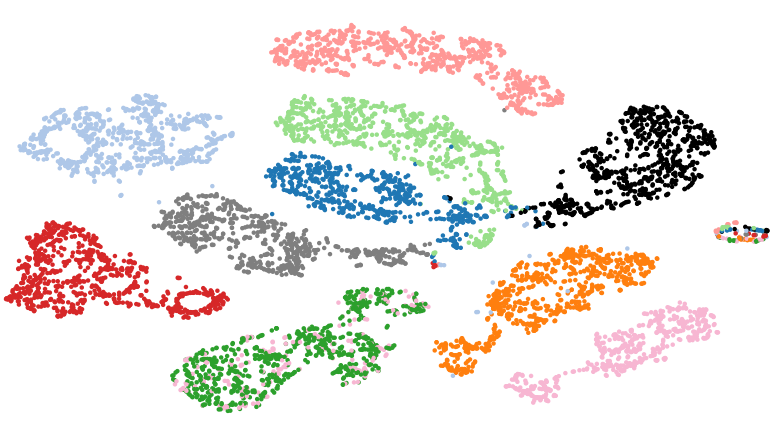$$t$$-SNE visualization of speaker representations learned by Contrastive Predictive Coding where each color represents a different speaker. Source: van den Oord et al. (2018).

Contrastive Predictive Coding, despite its sequential nature, can also be used to build representations from images. The latent representations at each timestep correspond to convolutions applied to a local patch of the image, where all the patches generally overlap.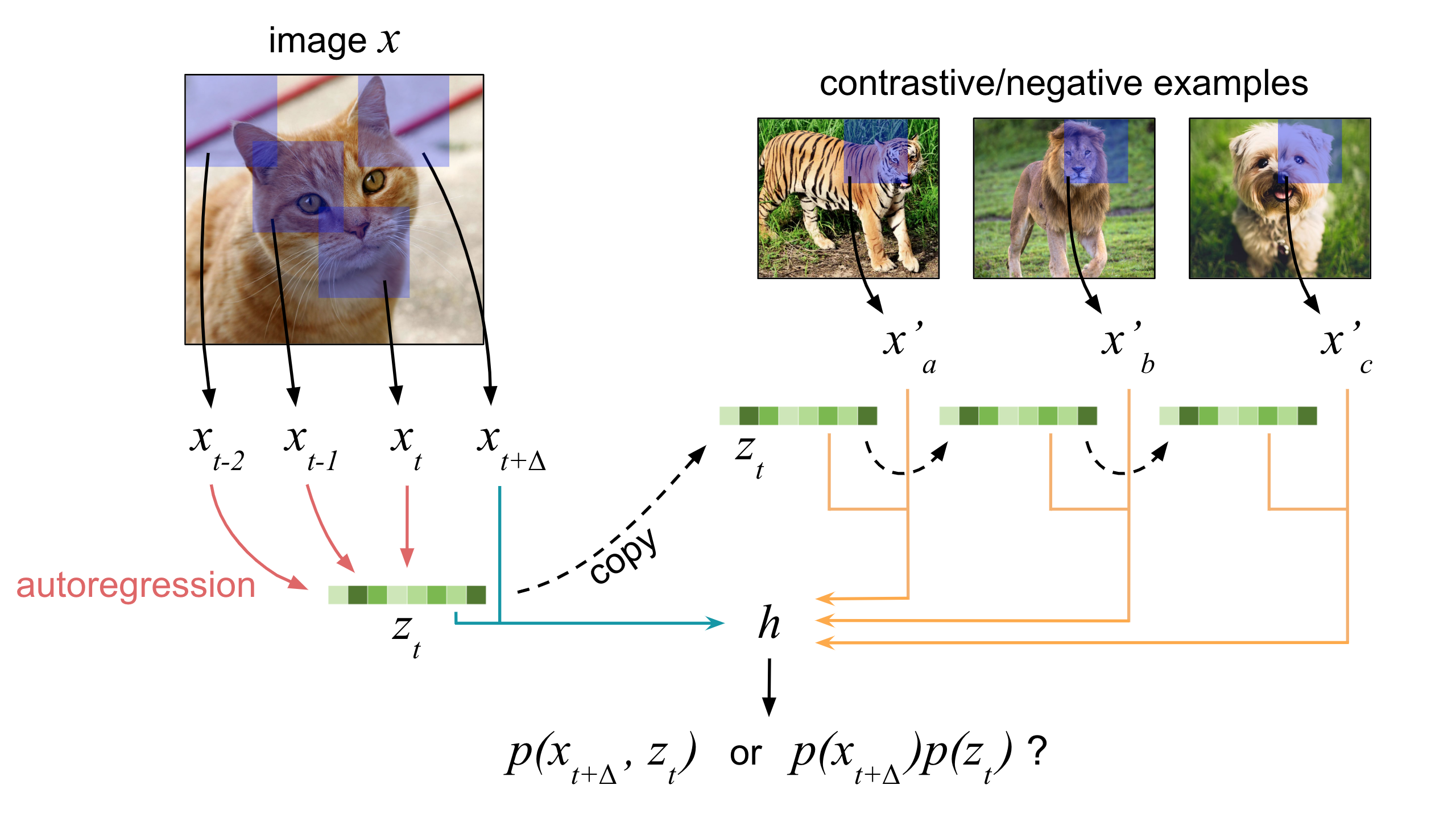Contrastive Predictive Coding for building representations from images. The latent representation at each time step is the feature map extracted from part of the image. These are used to construct a summary'' representation via autoregression. The mutual information between the summary representation and future latent representations is maximized by maximizing the contrastive lower bound.

#### Some Applications of the Contrastive Lower Bound

Unfortunately, learned representations can often capture noisy attributes that aren't really helpful. For instance, in the image domain, this may correspond to lighting, the angle from which picture was taken, etc. Ideally, we want to build representations of images that are invariant to all these noisy factors and focus solely on extracting the signal, such as what's actually in the image and wouldn't change even if the lighting of angle of the picture changes.

How can we do this? If we compare multiple images of the same object from different viewpoints, then they typically share some "information" which we may assume carries the signal we're interested in, while the rest corresponds to noise. Consequently, if we try to learn representations from all these separate viewpoints while simultaneously maximizing mutual information between them, we force the encoding process to eliminate the noise! Using the contrastive lower bound, the training objective is

$\max \mathbb{E}_{z_1^i, z_2^i} \left[ \log \frac{ h(z_1^i, z_2^i) }{ \sum_{j} h(z_1^i, z_2^j) } \right]$

where subscripts indicate viewpoint and superscripts index examples. This approach to maximizing mutual information about objects and scenes from different viewpoints/modalities was proposed simultaneously as Augmented Multiscale Deep InfoMax by one group of researchers at Microsoft Research and Contrastive Multiview Coding by another group at MIT and Google AI. They were both able to surpass AlexNet classification accuracies on ImageNet using the learned representations--that's an impressive feat considering the representations were learned in an unsupervised way!

This idea can generalize beyond the image domain. If we want to build representations of something which occurs multiple times in our dataset such that each sample contains lots of noise, we can maximize mutual information between learned representations from different encodings. In the audio domain, we can capture speaker representations that are invariant to factors such as microphone quality, background noise, etc.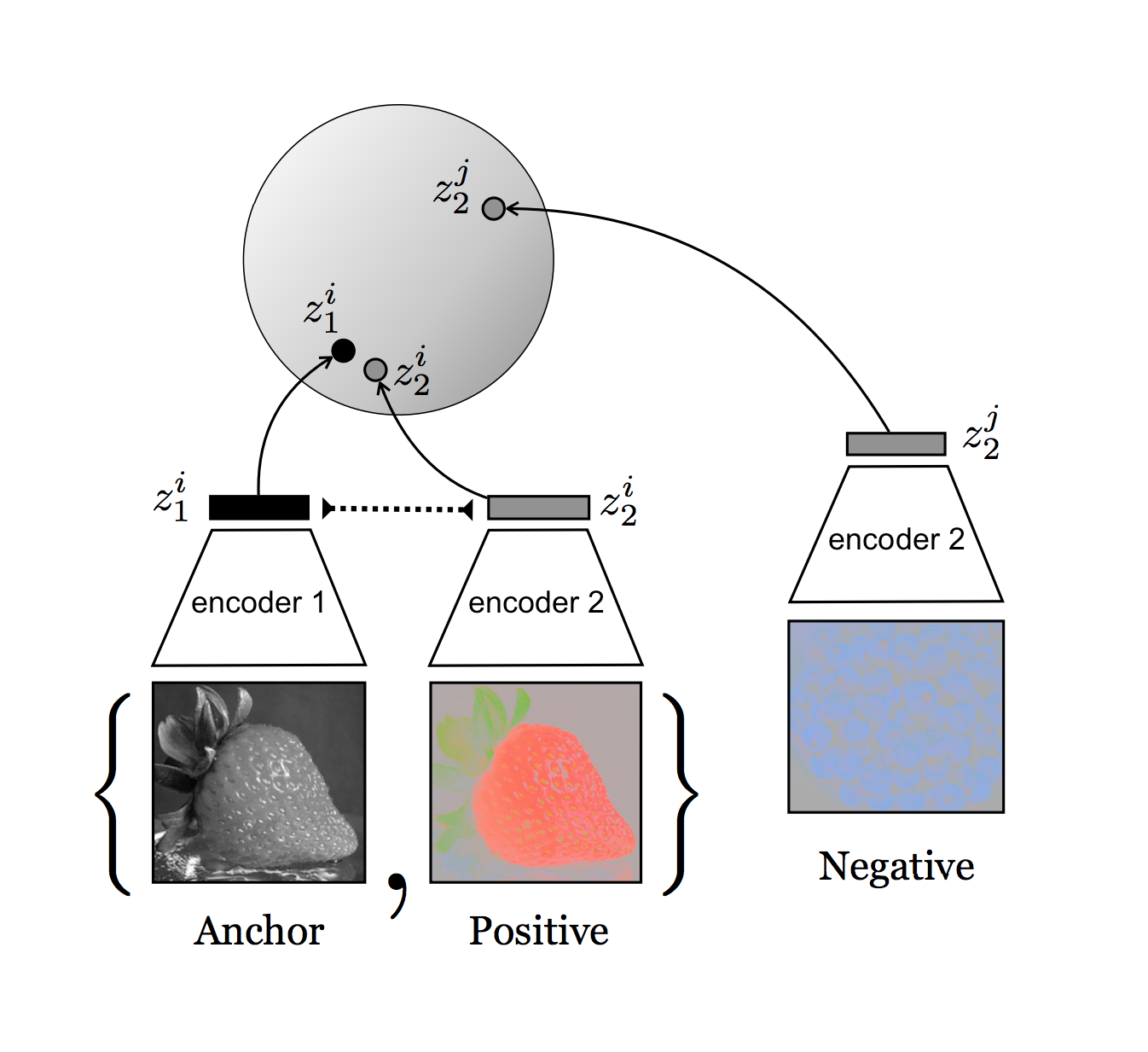The general framework behind Augmented Multiscale Deep InfoMax and Contrastive Multiview Coding. Multiple viewpoints of the same objects are encoded, and the mutual information between the encoded representations is maximized to obtain viewpoint-invariant representations. Source: Tian et al. (2019).

The last bound that we'll discuss is actually a lower bound on KL-divergences. Since mutual information is by definition the KL-divergence between $$p(x, z)$$ and $$p(x) p(z)$$, it also comes with a lower bound for free--how useful!

We'll start by formulating this lower bound: let $$q$$ be some arbitrary distribution over both $$x$$ and $$z$$. Then,

\begin{align*} I(x; z) &= D_\text{KL} \left( p(x, z) || p(x)p(z) \right) \\ &= \mathbb{E}_{p(x, z)} \left[ \log \frac{p(x, z)}{p(x)p(z)} \right] \\ &= \mathbb{E}_{p(x, z)} \left[ \log \left( \frac{q(x, z)}{p(x)p(z)} \frac{p(x, z)}{q(x, z)} \right) \right] \\ &= \mathbb{E}_{p(x, z)} \left[ \log \frac{q(x, z)}{p(x)p(z)} \right] + \underbrace{ D_\text{KL} \left( p(x, z) || q(x, z) \right) }_{\geq 0} \\ &\geq \mathbb{E}_{p(x, z)} \left[ \log \frac{q(x, z)}{p(x)p(z)} \right] \end{align*}

where we just multiplied and divided the term inside the $$\log$$ by $$q(x, z)$$ in the second line. Note that the inequality in the last line holds once again since KL-divergences are always non-negative. This bound is tight (which means that "$$\geq$$" becomes "$$=$$") whenever $$q(x, z) = p(x) p(z)$$, so we can write

$D_\text{KL}\left( p(x, z) || p(x) p(z) \right) \geq \sup_q \mathbb{E}_{p(x, z)} \left[ \log \frac{q(x, z)}{p(x)p(z)} \right] \\$

where $$\sup$$ refers to supremum (or asymptotically highest attainable) value of the expression over all possible forms of $$q$$. So good so far, right? Now we can actually decide what $$q$$ should be! Suppose we choose to parameterize it as

$q(x, z) = \frac{1}{K} p(x) p(z) e^{T_\theta(x, z)} = \frac{p(x) p(z) e^{T(x, z)}}{\int \int p(x') p(z') e^{T_\theta(x', z')} dz' dx'} \\$

where $$T_\theta$$ is a function over $$x$$ and $$z$$ that maps to a real number, parameterized by $$\theta$$, and $$K$$ is a normalizing term. Why choose this seemingly random form? Because it just works out nicely in the end--don't worry too much about it! Here, we also need to divide the numerator by $$K$$, whose job is to ensure $$q$$ remains a proper probability distribution that sums to 1. We can also write $$K$$ as

\begin{align*} K &= \int \int p(x') p(z') e^{T_\theta(x', z')} dx' dz' \\ &= \mathbb{E}_{p(x) p(z)} \left[ e^{T_\theta(x, z)} \right] \end{align*}

since this is just the definition of expected value. Substituting $$q$$ into the lower bound we just developed on the KL-divergence,

\begin{align*} D_\text{KL} \left( p(x, z) || p(x)p(z) \right) &\geq \sup_\theta \mathbb{E}_{p(x, z)} \left[ \log \frac{p(x) p(z) e^{T_\theta(x, z)}}{K p(x) p(z)} \right] \\ &= \sup_\theta \mathbb{E}_{p(x, z)} \left[ \log \frac{ e^{T_\theta(x, z)} }{ \mathbb{E}_{p(x) p(z)} \left[ e^{T_\theta(x, z)} \right] } \right] \\ &= \sup_\theta \mathbb{E}_{p(x, z)} \left[ T_\theta(x, z) \right] - \log \mathbb{E}_{p(x) p(z)} \left[ e^{T_\theta(x, z)} \right] \end{align*}

and we're done! This is referred to as the Donsker-Varadhan representation of the KL-divergence. This bound was used by researchers at Mila to estimate the mutual information between two variables using a neural network.

Moreover, since the Donsker-Varadhan representation is a lower bound on mutual information (by virtue of being a lower bound on the KL-divergence), it can also be used as an objective to learn representations. Deep InfoMax, formulated by researchers at Mila and Microsoft Research, does precisely this. An encoder maps data samples $$x$$ to representations $$z$$ and $$T_\theta$$, parameterized by--you guess it--a neural network, acts as a discriminator that tells samples drawn from $$p(x, z)$$ apart from those drawn from $$p(x) p(z)$$. This in turn forces the encoder to generate representations that have high mutual information with the original data (because the encoder tries to maximize the Donsker-Varadhan representation and thus mutual information). Deep InfoMax's representations outperform representations learned by various other popular unsupervised algorithms for downstream image classification tasks on many benchmark image datasets.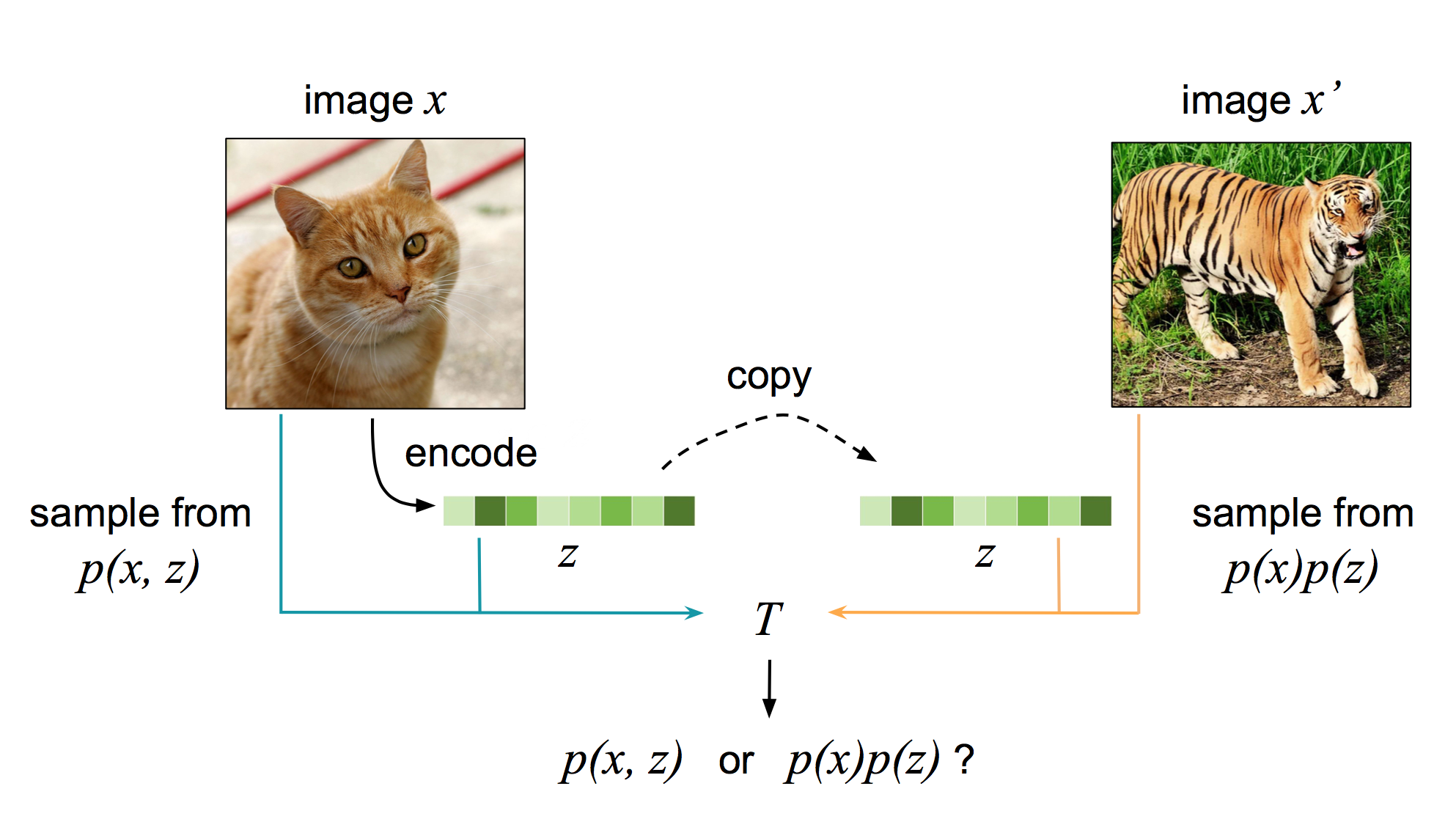Framework for Deep InfoMax, which optimizes the Donsker-Varadhan representation of the KL-divergence to maximize mutual information between inputs and their learned representations. The left half of the figure gives a data-representation sample drawn from $$p(x, z)$$ whereas the right half gives one from $$p(x) p(z)$$. $$T_\theta$$'s job is to tell them apart, which forces the encoder to maximize mutual information between data and their representations.

#### Recap

Now that we've gotten through all the math and derivations, let's quickly review the takeaway points. First, we talked about the variational lower bound on mutual information. This requires another neural network $$q_\theta(z|x)$$ with parameters $$\theta$$ to approximate the true posterior distribution over representations, $$p(z|x)$$. The second bound was the contrastive lower bound which requires many datapoints to be contrasted with a single representation in order to maximize mutual information between the true datapoint correspond to that representation and the representation itself. The third bound, similar in idea to the contrastive lower bound, is the Donsker-Varadhan representation of the KL-divergence which is essentially a lower bound on mutual information since its a lower bound on KL-divergences.

#### Final Words

If you're reading this, you've made it all the way to the end of this article--thanks for reading! Mutual information-based objectives in unsupervised learning have been showing promising results, and there is still lots of work left to be done with useful applications. Feel free to contact me if you have any questions or comments.

A special thanks to Max Smith for providing feedback on this article.

#### References

1. Alemi, Fischer, Dillon, Murphy. Deep Variational Information Bottleneck. 2017.
2. Bachman, Hjelm, Buchwalter. Augmented Multiscale Deep Infomax. 2019.
3. Belghazi, Baratin, Rajeswar, Ozair, Bengio, Courville, Hjelm. Mutual Information Neural Estimation. 2018.
4. Chen, Duan, Houthooft, Schulman, Sutskever, Abbeel. InfoGAN. 2016.
5. Hjelm, Fedorov, Lavoie-Marchildon, Grewal, Bachman, Trischler, Bengio. Deep InfoMax. 2019.
6. Ravanelli and Bengio. Learning Speaker Representations with Mutual Information. 2018.
7. Tian, Krishnan, Isola. Contrastive Multiview Coding. 2019.
8. van den Oord, Li, Vinyals. Contrastive Predictive Coding. 2019.